CH3-CH2-CH2-C$\equiv$CH+LiHN2$\to \left(\mathrm{A}\right)\stackrel{\left({\mathrm{CH}}_{3}{\right)}_{2}{\mathrm{SO}}_{4}}{\to }$(B)

Give the structural formula of compound (B):

(a) CH3-(CH2)2-C$\equiv$C-SO3H

(b) CH3-(CH2)2-C$\equiv$C-CH3

(c)  CH3-(CH2)2-C$\equiv$C-CH2-O-$\begin{array}{c}\stackrel{\mathrm{O}}{\parallel }\\ \mathrm{s}\\ \underset{\mathrm{O}}{\parallel }\end{array}$-H

(d) CH3-CH2-C$\equiv$C-CH2

High Yielding Test Series + Question Bank - NEET 2020

Difficulty Level: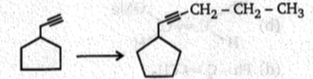; This conversion can be achieved by: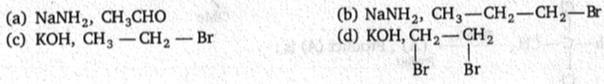High Yielding Test Series + Question Bank - NEET 2020

Difficulty Level:

Which alkyne will give 3-ethylhexane on catalytic hydrogenation?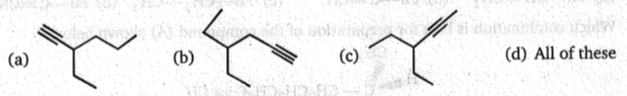High Yielding Test Series + Question Bank - NEET 2020

Difficulty Level:

Lindlar
Br-(CH2)12-C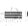CH
(A)(B);Product (B) is :
NaNH2   Catalyst
1.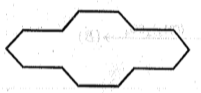2.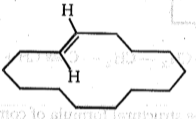3.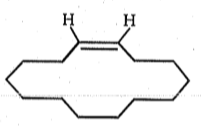4.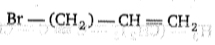High Yielding Test Series + Question Bank - NEET 2020

Difficulty Level:

Ph - C $\equiv \mathrm{CH}\underset{\mathrm{MeOH}}{\overset{{\mathrm{MeO}}^{-}}{\to }}$ Major product of the reaction is:

(a)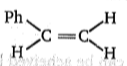(b)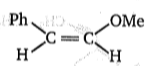(c) Ph - C $\equiv$C-OMe

(d)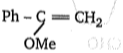High Yielding Test Series + Question Bank - NEET 2020

Difficulty Level:

; Product (A) is:

(a) Ph-CH=CH2          (b) Ph-C$\equiv$CH              (c) Ph-CH2-CH3             (d) Ph-C$\equiv$$\stackrel{⊝}{\mathrm{C}}\stackrel{\oplus }{\mathrm{Na}}$

High Yielding Test Series + Question Bank - NEET 2020

Difficulty Level: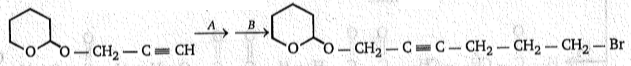To carry out above conversion, (A) and (B) respectively, are :

(a) NaNH2, Cl - CH2ーCH- CH- Br
(b) NaNH2, CH2-CH2-CH2-Br
(c) NaNH2, l-CH2ーCH2-CH2-Br
(d) NaNH2, I-CH2-CH2-CH2-I

High Yielding Test Series + Question Bank - NEET 2020

Difficulty Level:

H-C$\equiv$C-Ph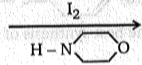Product; Product obtained in this reaction is:

(a) $\begin{array}{ccccccc}\mathrm{Ph}& -& \underset{\mathrm{I}}{\underset{|}{\mathrm{C}}}& =& \mathrm{CH}& -& \begin{array}{c}\mathrm{I}\end{array}\end{array}$

(b) $\begin{array}{ccccccc}\mathrm{PH}& -& \underset{\mathrm{I}}{\underset{|}{\mathrm{CH}}}& -& {\mathrm{CH}}_{2}& -& \mathrm{i}\end{array}$

(c) Ph-C$\equiv$C-I

(d) I-C$\equiv$C-H

High Yielding Test Series + Question Bank - NEET 2020

Difficulty Level: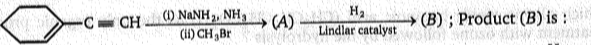(a)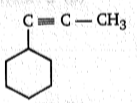(b)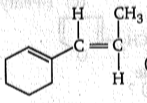(c)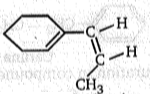(d)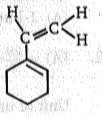High Yielding Test Series + Question Bank - NEET 2020

Difficulty Level:

Which of the following alkyne on treatment with H2(2 mole)/ Pt gives an optically inactive compound ?

(a) 3-Methyl-1-pentyne

(b) 4-Methyl-1-hexyne

(c) 3-Methyl-1-heptyne

(d) None of the above